Image by pangziai on Pixabay

## 什么是“树”？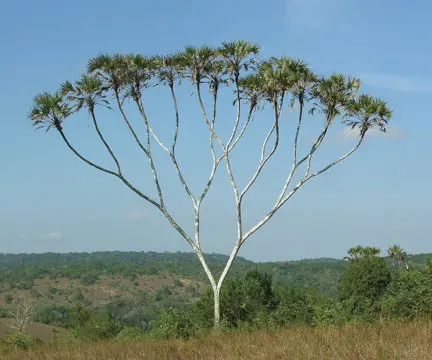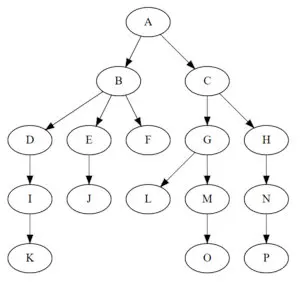## 二叉树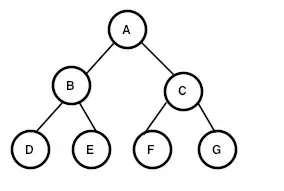### 常见术语

• 结点（node）：上图中的 A、B、C。。。就是一个结点
• 结点的度：一个结点含有的子树的个数称为该结点的度
• 树的度：一棵树中，最大的结点的度称为树的度
• 深度：对于任意节点 n , n 的深度为从根到 n 的唯一路径长，根的深度为 0；
• 高度：对于任意节点 n , n 的高度为从 n 到一片树叶的最长路径长，所有树叶的高度为 0；

### 种树

1. 根结点
2. 左子树和右子树

``````class TreeNode {
String data;
TreeNode left;
TreeNode right;

TreeNode(String data) {
this.data = data;
}
}
``````

``````// 创建二叉树
private TreeNode createTree() {
TreeNode root = new TreeNode("A");
root.left = new TreeNode("B");
root.right = new TreeNode("C");
root.left.left = new TreeNode("D");
root.left.right = new TreeNode("E");
root.right.left = new TreeNode("F");
root.right.right = new TreeNode("G");
return root;
}
``````

### 树的特征

• 判断是否为空
• 获取树的高度
• 获取树中的结点个数

#### 判断是否为空

``````// 判断是否为空
public boolean isEmpty(TreeNode root) {
return root == null;
}
``````

#### 获取树的高度

``````// 获取树的高度
private int height(TreeNode root) {
if (root == null)
return 0;//递归结束：空树高度为0
else {
int i = height(root.left);
int j = height(root.right);
return (i < j) ? (j + 1) : (i + 1);
}
}
``````

#### 获取树的结点大小（个数）

``````// 获取结点大小
private int size(TreeNode root) {
if (root == null) {
return 0;
} else {
return 1 + size(root.left) + size(root.right);
}
}
``````

### 遍历二叉树

• 访问结点本身（N）
• 访问左结点（L）
• 访问右结点（R）``````前序：A B D E C F G

``````

#### 前序遍历

``````// 前序遍历二叉树
private void preOrder(TreeNode root) {
if (root != null) {
System.out.print(root.data + " ");
preOrder(root.left);
preOrder(root.right);
}
}
``````

#### 中序遍历

``````// 中序遍历二叉树
private void inOrder(TreeNode root) {
if (root != null) {
inOrder(root.left);
System.out.print(root.data + " ");
inOrder(root.right);
}
}
``````

#### 后序遍历

``````// 后序遍历二叉树
private void postOrder(TreeNode root) {
if (root != null) {
postOrder(root.left);
postOrder(root.right);
System.out.print(root.data + " ");
}
}

``````

#### 层序遍历

``````// 层序遍历
private void levelTravel(TreeNode root) {
if (root == null) return;
while (!q.isEmpty()) {
TreeNode temp = q.poll();
System.out.print(temp.data + " ");
}
}

``````

## 扩展

### 二叉树的作用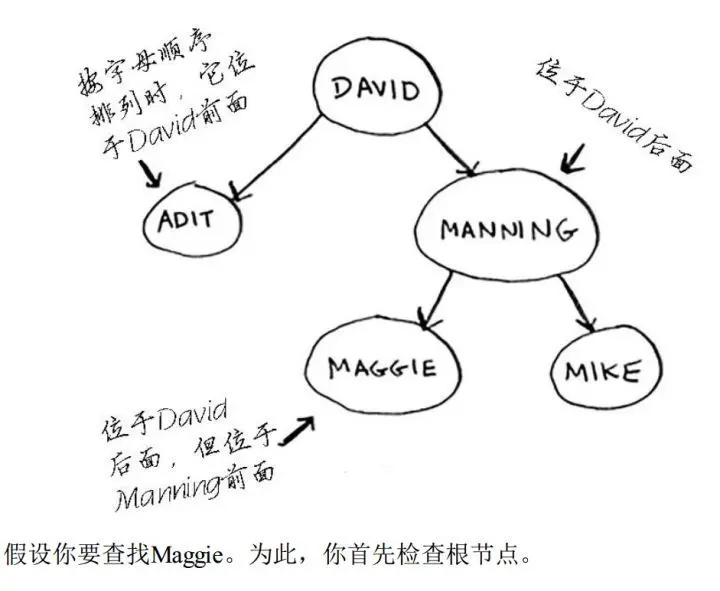`Maggie`排在`David`后面，因此向右找`Maggie`，排在`Manning`前面，因此向左找。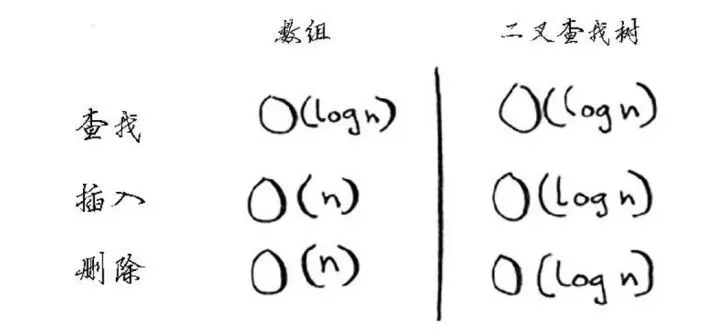### 二叉树的扩展

• B- 树，是一种特殊的二叉树，数据库常用它来存储数据。
• B+ 树，B+树是 B-树的一种变体，主要用于磁盘文件组织、数据索引和数据库索引等场景。
• 红黑树，二叉平衡树的一种，Java 中的 TreeSet ,TreeMap，HashMap 就是这种数据结构。
• 堆，是一种完全二叉树，可以实现优先队列。

## END

“关注我最好的时间是 3 分钟前，而后是现在 👇”